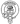# Automatic Semi-colon Insertion in JavaScript

August 12, 2020

I’m a big fan of prettier and have been using it in every project I’ve started over the past few months, it’s an opinionated code formatter that I have configured to format my code whenever I save it. Recently I decided to try adjusting my rule set to no longer enforce semi-colons at the end of each statement.

At first all seemed to be well and I felt my code was was more readable and my brain was under less cognitive load. However while trying to write a function to swap the position of two elements in an array I came across something which I initially believe to be a formatting bug but turned out to be something far more interesting.

I had the following code snippet:

``````const swap = (array, a, b) => {
[array[a], array[b]] = [array[a], array[b]]
}``````

But when I hit save prettier formatted the code to this:

``````const swap = (array, a, b) => {
;[array[a], array[b]] = [array[a], array[b]]
}``````

I hit the docs and soon enough came across a section explaining what setting the semi-colon option to false really does.

false - Only add semicolons at the beginning of lines that may introduce ASI failures.

This peaked my interest, what were ASI failures and what are the rules that govern them? First let’s start with what ASI is, unlike other C-like languages, JavaScript does not enforce the use of a semicolon at the end of a statement. Instead the JavaScript interpreter will add them when it runs the code. So where does it add them?

The rules the interpreter uses to automatically insert semi-colons can be broken down in plain english as the following:

1. two statements are separated by a line terminator
2. two statements are separated by a closing brace (’}‘)
3. a line terminator follows a break, continue, return, or throw.

Great! So we can just leave our semi-colons out and let the interpreter do all the heavy lifting right? Well sort-of turns out there’s quite a few gotchas and situations where ASI can actually cause you problems. Take a look at these examples:

``````// Original Code
i
++;

// Interpreted As
i;
++;

// Correct Code
i++;``````
``````// Original Code
const exampleFunc = () => {
return
{
// more code
};
}

// Interpreted As
const exampleFunc = () =>  {
return;
{
// more code
};
}

// Correct Code
const exampleFunc = () =>  {
return {
// more code
};
}``````
``````// Original Code
return
1 * i + 5;

// Interpreted As
return;
1 * i + 5;

// Correct Code
return 1 * i + 5;``````
``````// Original Code
if (i === 5)
// assuming a semicolon here
else
foo = 0;

// Interpreted As
if (i === 5)
// no semicolon here
else
foo = 0;

// Correct Code
if (i === 5){
// more code
} else {
foo = 0;
}``````

So back to my original example why is prettier adding the semi-colon at the start of my statement? Well it’s actually a preventative measure. Currently the code would run fine without the semi-colon but what if we were to add another line so that this:

``````const swap = (array, a, b) => {
[array[a], array[b]] = [array[a], array[b]]
}``````

``````const swap = (array, a, b) => {
console.log('Hello World!')
[array[a], array[b]] = [array[a], array[b]]
}``````

Well then our code would actually mean:

``````const swap = (array, a, b) => {
console.log('Hello World!')[array[a], array[b]] = [array[a], array[b]]
}``````

By prepending our original line with a semi-colon prettier was actually trying to help us! By doing so we not longer have to juggle the ASI rules we learnt earlier in our head while we code and can instead let it do all the heavy lifting for us.Written by Fraser Hamilton a full stack developer based out of Edinburgh, Scotland.